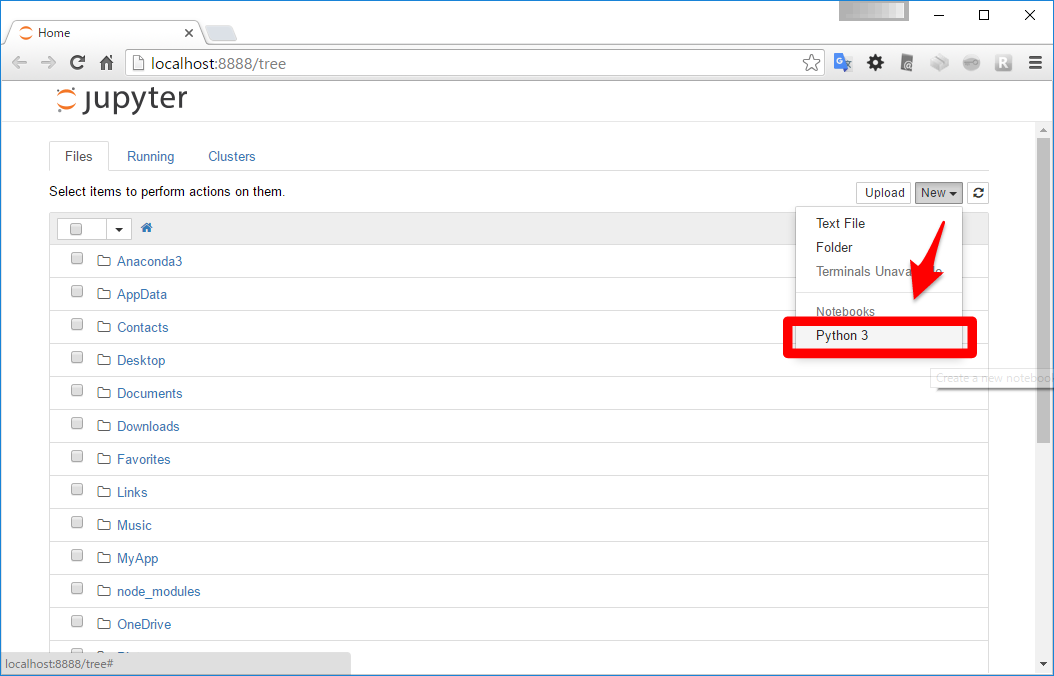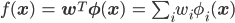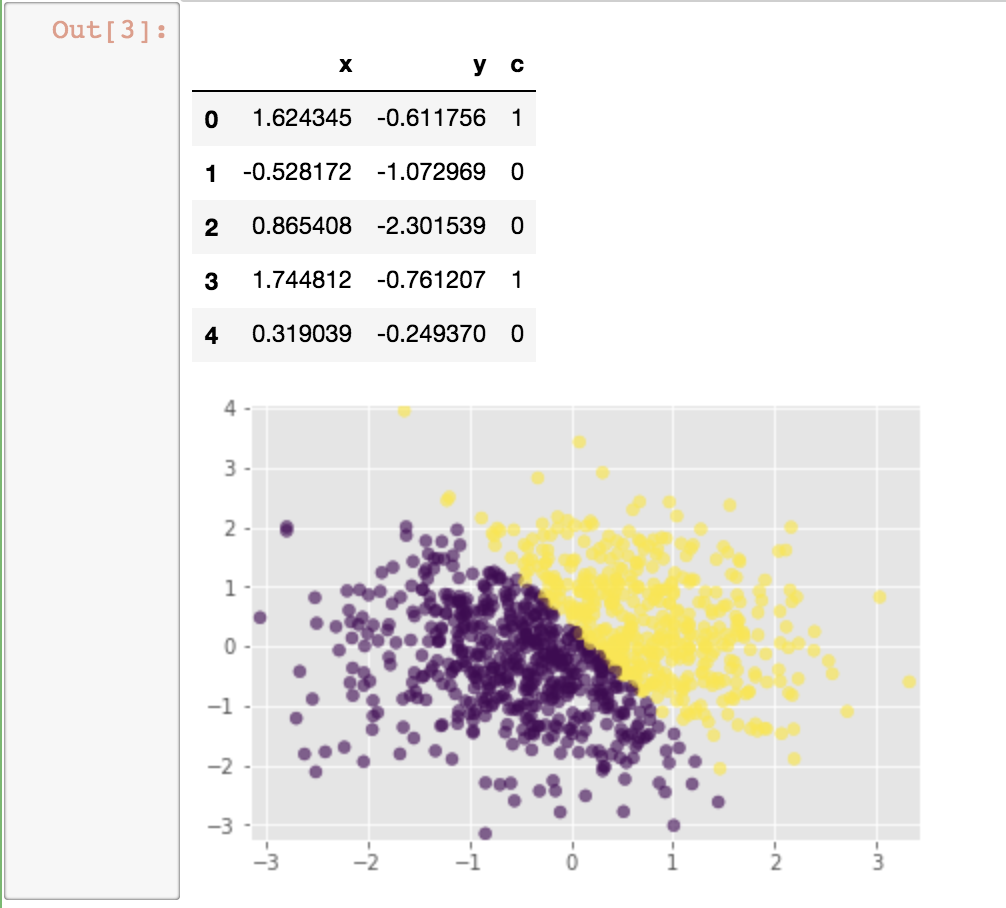# Data Science

## Start learning data science.

• Necessary to download Anaconda first.
• Execute `jupyter notebook` in teminal.
• Select Python3 in jupyter notebook.*Refered from other site.

## How to visualize data

• Necessary to code `%matplotlib inline at the first line.`
• All necessary process has been done. Now you can keep codeing like whatever you want to code.

## What is logistic regression model?

It's much easier to understand what logistic regression model is with thinking about a classifier of a spam e-mail. Basically, a spam e-mail filter classify which is a spam or not. There would be some experiences like an e-mail should be classified as a spam got to through the filter. On the other hands,an email should be classified it's not a spam got to classify as a spam. So we need to take steps to predict with deep learning. Classifying it's not a spam when program thinks the prediction is weak. This is one of methods to reduce the bad possibility such as classifying an e-mail as a spam. For doing this,we need to measure how reliable the prediction is.

## Review of perceptron.*Refered from other site.

Frankly to say,perceptron is a binary classifier which predicts which kind of data points with classifying f(x) is plus or minus.

## Generating visualized data.

```>|python|
%matplotlib inline

import numpy as np
import pandas as pd
import matplotlib.pyplot  as plt
from scipy import optimize

plt.style.use('ggplot')

def make_data(N, draw_plot=True, is_confused=False, confuse_bin=50):
'''N個のデータセットを生成する関数
データをわざと複雑にするための機能 is_confusedを実装する
'''
np.random.seed(1) # シードを固定して、乱数が毎回同じ出力になるようにする

feature = np.random.randn(N, 2)
df = pd.DataFrame(feature, columns=['x', 'y'])

# 2値分類の付与：人為的な分離線の上下どちらに居るかで機械的に判定
df['c'] = df.apply(lambda row : 1 if (5*row.x + 3*row.y - 1)>0 else 0,  axis=1)

# 撹乱:データを少し複雑にするための操作
if is_confused:
def get_model_confused(data):
c = 1 if (data.name % confuse_bin) == 0 else data.c
return c

df['c'] = df.apply(get_model_confused, axis=1)

# 可視化：どんな感じのデータになったか可視化するモジュール
# c = df.c つまり2値の0と1で色を分けて表示するようにしてある
if draw_plot:
plt.scatter(x=df.x, y=df.y, c=df.c, alpha=0.6)
plt.xlim([df.x.min() -0.1, df.x.max() +0.1])
plt.ylim([df.y.min() -0.1, df.y.max() +0.1])

return df

df = make_data(1000)
df.head(5)
||<
```